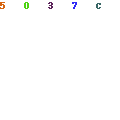# Introduction to Gradient Boosting ClassifierI graduated in Computer Science a few days ago, so now I have finally time to writing again here. Some time ago I wrote an article about Adaptive Boosting Classifier. Today I would like to introduce you to another ensemble classifier – Gradient Boosting Classifier. Here we are dealing with the creation of a prediction model in the form of a set of weak models as well.

Theoretical introduction

Gradient Boosting Classifier is a classifier that produces a prediction model in the form of a set of weak models, which are usually in the form of decision trees. Successive trees are generated during the learning process. This algorithm builds the first model to predict the value and calculate the loss, which is the difference between the first model’s result and the actual value. A second model is then built to predict the loss after the first step. This process continues until a satisfactory result is achieved.
The main idea behind gradient boosting is to iteratively find new trees that minimize the loss function. The loss function is a measure of how large the errors your model makes. The input of the algorithm is the training set \lbrace {(x_i,y_i) }\rbrace ^{n}_{i=1} , the loss function L(y, F(x)) function and the number of iterations M.

The algorithm can be presented in the following steps:

1. Initialization of the model in the form of constant:

2. For m = 1 to M .

a) Calculation of the negative gradient for i = 1, …, n :

b) Matching a learner h_m(x) to a pseudo-remainder, i.e. training him with the training set \lbrace {(x_i,r_i) }\rbrace ^{n}_{i=1} .

c) Calculation of the multiplier \tau_m by solving the optimization problem:

d) Updating the model:

3. Output F_m(x) .

Example in Python

If you want to use this algorithm in Python, you can do that with the sklearn library. Just import the following method:

from sklearn.ensemble import GradientBoostingClassifier

Let me leave part of the implementation responsible for preparing the training and test set. I will proceed to use the classifier:

gbc = GradientBoostingClassifier()
gbc.fit(x_train, y_train.ravel())
predictions = gbc.predict(x_test)

As I mentioned in earlier articles, the library allows you to set certain parameters for the classifier, such as the depth of individual estimators or tolerance for premature stopping of the process. All information can be found in the documentation.

Summary

I wanted to introduce you to Gradient Boosting Classifier. This is another ensemble classifier I wrote about here. If you want to read more on this subject, I encourage you to check the book Combining Pattern Classifiers Methods and Algorithms by Ludmila I. Kuncheva. I invite you to read my other articles connected with Machine Learning.

Sources

 Leo Guelman, Gradient boosting trees for auto insurance loss cost modeling and prediction, Expert Systems with Applications Volume 39, Issue 3, Pages 3659-3667, 2012

## 1 Comment

1.What a really awesome post this is. Truly, one of the best posts I’ve ever witnessed to see in my whole life. Wow, just keep it up.